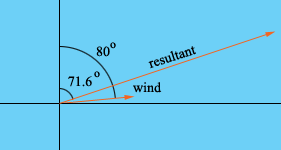SEARCH HOMEMath Central Quandaries & QueriesQuestion from Maria, a parent: The aeroplane flies in a wind of speed 50 kph from the direction S80◦W. However, the direction in which it is pointed, and its speed in still air, are such that its resultant speed and direction are 800 kph, and direction N71.6◦E I need to find the size of the angle between v and vw, in degrees to one decimal place, and find the speed at which the aeroplane would fly if the air were still, to the nearest kph. It would help me greatly if I could see a diagram illustrating the velocity va that the aeroplane would have if the air were still, the velocity vw of the wind, and the resultant velocity v of the aeroplane (diagram should be in the form of a triangle illustrating how one velocity vector is the sum of the other two) ThanksHi Maria,

Since the wind is from the direction S80oW the wind vector is in the direction N80oE. Hence my diagram isHence the vector representing the direction and length of the aeroplane in still air must be from the end of the wind vector to the end of the resultant vector.

I hope this helps,
HarleyMath Central is supported by the University of Regina and The Pacific Institute for the Mathematical Sciences.